# Newton's Laws and the Physics of Flight

Andrew Wood | 20 July 2023

In this post we examine the fundamental physics laws governing heaver-than-air flight. Specifically, we'll look at the relationship between mass, force, and acceleration through Newton's Laws, and how this affects the performance characteristics of an aircraft. These principles form a basis of understanding of the motion of any body with mass on Earth and so are directly applicable to operating a light aircraft.

## Air is a Fluid

While it may seem counterintuitive, air is in fact a fluid with all the associated properties:

• Air is viscous and flows like a liquid.
• Air exerts a pressure on any body it surrounds.
• There is friction between air molecules.

### Pressure

Pressure is the force per unit area applied perpendicular to the surface of an object. A stationary object immersed in a fluid is subjected to a uniform pressure at all points on the object. In the case of still air, the air pressure (static pressure) is best thought of as the weight of the air column pushing down on that object. Air has a density and therefore a mass. The weight of the air is simply the mass of the air multiplied by gravity, and the pressure is the distribution of that weight force over the area of the object.

At higher altitudes the mass of air pushing down on the object is less and as such the static pressure decreases as altitude increases. An altimeter simply measures the current static pressure and relates this to altitude.

### Viscosity

Viscosity is the resistance of a fluid (either a liquid or a gas) to deformation. This is best thought of in terms of the thickness or resistance to flow of a liquid. Syrup is highly viscous and therefore does not flow easily compared to less viscous water.

Since air is a fluid, it too has viscosity. There are internal frictional forces between adjacent layers of air that require a stress (pressure) to cause the air to flow. A pressure difference therefore exists between two regions of flow. Said another way, when pressure differences exist between two fluid regions, a force will be generated, resulting in fluid flow as the pressure tries to equalize.

### Friction

While the frictional forces between layers of air are very small, larger frictional forces are present at the boundary between the air and any contact surface. In the case of an airplane, there is friction between the air and the surface of the airframe. The air directly on the surface is brought to rest as those air molecules stick to the surface. A velocity gradient exists between the surface and some point just above the surface which we term the boundary layer.

This boundary layer causes a form of resistance to the motion of the aircraft, a term we call friction drag.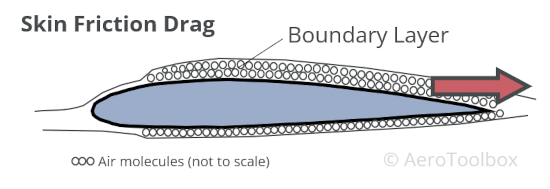## Newtons Laws

Sir Isaac Newton (1642 - 1726) formulated three laws governing motion known today as Newton's Laws. Understanding these three laws are crucial to understanding the basic physics behind how an aircraft flies.

Newton also formulated the law of universal gravitation, which describes how every particle in the universe attracts every other with a force proportional to the product of their masses and inversely proportional to the square of the distance between the particles. This explains the gravitational force we experience on Earth which always acts downwards, keeping us on the ground.

### Newtons First Law

A body at rest will remain at rest unless acted upon by an unbalanced force. A body moving at a constant velocity will continue to do so in a straight line unless acted upon by an unbalanced force.

This law describes how no mass will start or stop moving (accelerate or decelerate) unless some outside force causes it to do so. An airplane sitting on a level apron will not move unless sufficient engine thrust is produced to overcome the aircraft's inertia. Likewise, an aircraft flying at a constant speed will not accelerate unless the thrust is increased such that thrust is greater than drag.

Inertia refers to the resistance of any physical body to a change in its velocity and is one of the primary manifestations of mass.

### Newtons Second Law

When a force is applied to a body, the magnitude of the force is equal to the time rate of change of its momentum.

For a constant mass, the force is equal to the mass of the body multiplied by its acceleration:

$$F = M \times a$$

Momentum is defined as the product of the mass and velocity of a body. When the mass is constant, the rate of change of momentum is solely a function of the rate of change of the velocity. The rate of change of velocity is termed acceleration.

### Newtons Third Law

When two bodies exert a force on each other, the force will be equal in magnitude but opposite in direction.

This is more commonly described as for every action there is an equal and opposite reaction.

The movement of a propeller through the air pushes the air backwards over the aircraft. Consequently, the air pushes the propeller (and the rest of the aircraft) forward.

## Newton's Laws Applied to Flight

We can apply Newtons Laws directly to an aircraft in flight in order to show how balanced or unbalanced forces affect an aircraft's position, velocity and acceleration.

### Four Fundamental Forces

An aircraft has mass and is subjected to gravity; thus it has a weight. This weight acts vertically downward at the center of gravity. In order to get airborne an aircraft must produce an upward force to counteract the weight. We call this force lift. Lift is produced by airflow over the wings and is always measured normal to the relative airflow.

Aerodynamic drag is the force that resists motion through the air. Similar to how water impedes a swimmer, air impedes the motion of the aircraft. To overcome this drag requires a thrust force which is produced by an engine. There are therefore four fundamental forces acting on an aircraft: thrust and drag, lift and weight.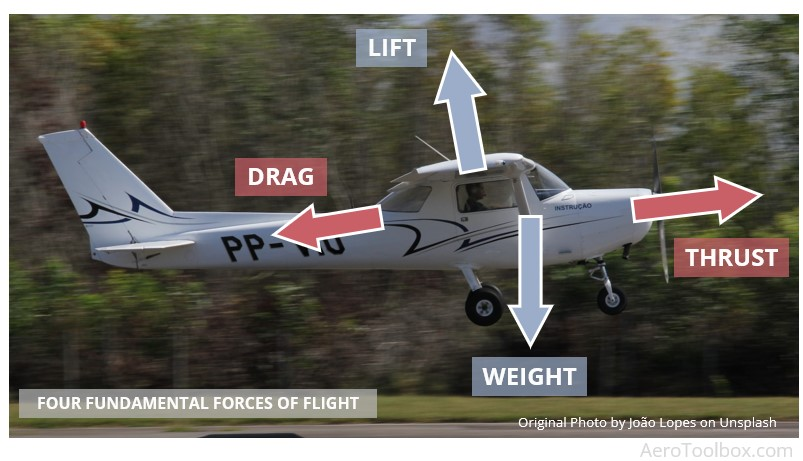### Straight and Level Flight

An aircraft flying straight and level at a constant speed is not accelerating in any direction meaning no net force can be acting in any direction. Therefore, the four fundamental forces must balance each other out. Assuming that the lift and weight act through the same point (they generally don't - which is why you need a horizontal tail), and that the line of action between the thrust and drag are the same (seldom the case), you can simplify the force balance to show that thrust is equal to drag and lift is equal to weight in straight and level flight at a constant speed.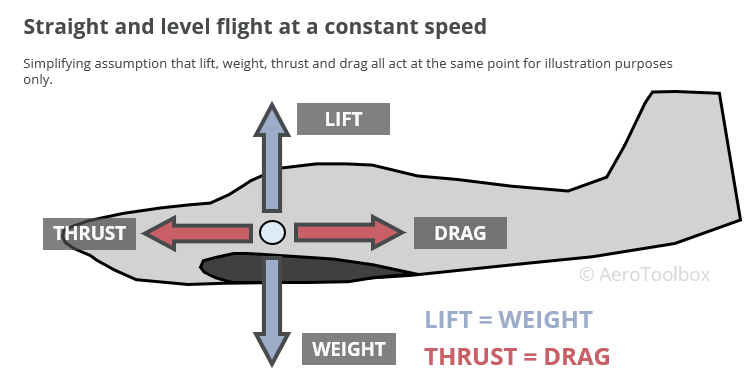### Accelerating Level Flight

In this case the thrust would need to be greater than the drag in order that an unbalanced horizontal force be applied to the aircraft. Based on our discussion of Newton's Laws, the aircraft would begin to accelerate in the direction of the unbalanced force (accelerate forward and speed up). As the aircraft accelerates and the speed increases, the drag will correspondingly increase (by the square of the speed) until a new equilibrium condition is reached where the drag and thrust are once again equal.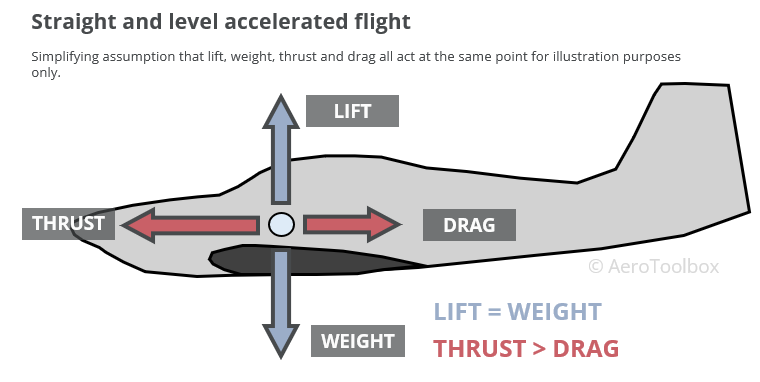### Climbing at a Constant Speed

Light aircraft are typically climbed at a constant speed; either the speed which produces the best rate of climb (Vy) or the best angle of climb (Vx). Since the speed is constant there is no acceleration and the forces acting on the aircraft must all be balanced.

How does the force balance look when the aircraft climbs? To answer this, we need to know a little about vectors

Two things characterise a constant speed climb:

1. A component of the total weight acts in the same direction as the drag force. This requires that additional thrust be produced to balance the longitudinal forces.
2. Weight always acts vertically down. This means that the total lifting force produced by the wing is less than the weight of the aircraft.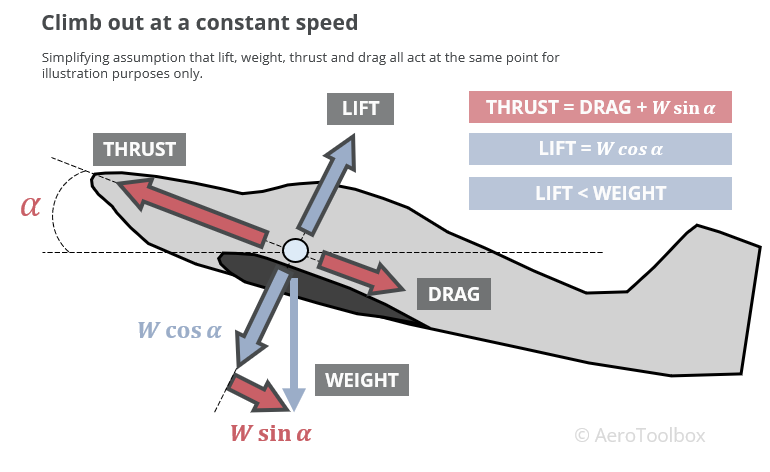You can refer to our article on Fundamental Forces in Flight for further information.

## Wrapping Up

Newton's three laws can be applied to an aircraft in motion to explain its current state.

• An aircraft at rest or moving at a constant velocity has no net force acting on it in any one direction. This means that all forces acting on the body must be balanced by an equal but opposite force (Newton 1).
• An aircraft will accelerate in the direction of the net force applied. Increasing the thrust produced by the engine will cause an acceleration in the direction of the applied thrust. Similarly, braking during the landing roll causes the aircraft to decelerate as the brake force is applied in the direction opposite to the motion of the plane (Newton 2).
• The movement of a propeller through the air forces accelerating air backwards over the aircraft. Consequently, the air pushes the propeller (and the rest of the aircraft) forward (Newton 3).
Share
Category
Tags